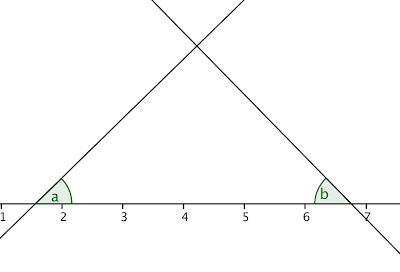## Thursday, April 2, 2009

### Neat proofs with tangents and slopes

Spring break is over, and it's time to do some math again!

Let's say you have two lines, y1 and y2, with unknown slopes. However, you do know that y1 is perpendicular to y2, like so:Without invoking the triangle sum, how can you prove two marked angles are complementary? With a simple use of the co-function.

If angles a and b are complementary, then a + b = 90, and 90 - a = b.

The co-function of tangent is co-tangent: cot (90 - a) = tan (a)*, so cot (b) = tan (a) if a and b are complementary. Is that true? To see, a few more labels help.Cot (b) is just x2/h, and tan (a) is just h/x1. So, is x2/h = h/x1? You bet -- the slope of lines are (negative) reciprocals of each other.

* Proving co-functions is simple. Starting with the unit circle:The coordinates of (x, y) = (cos (a), sin (a)). However, if you begin at (1, 0) and move clockwise (therefore, an angle of 90 - a), the points are a mirror image reflected over the y = x line of those before, and therefore (y, x) = (cos(90 - a), sin(90 - a). This defines the basic co-functions: x = cos(a) = sin(90 - a), y = sin(a) = cos(90 - a). And it follows that cot(90 - a) = cos(90 - a)/sin(90 - a) = sin(a)/cos(a) = tan(a).

#### 1 comment:

1."cot (90 - a) = tan (a)"

Proof of this identity requires knowledge of the fact that the two acute angle in a right triangle are complementary.

If it is unknown that a and b are complementary, then use of the identity is invalid.